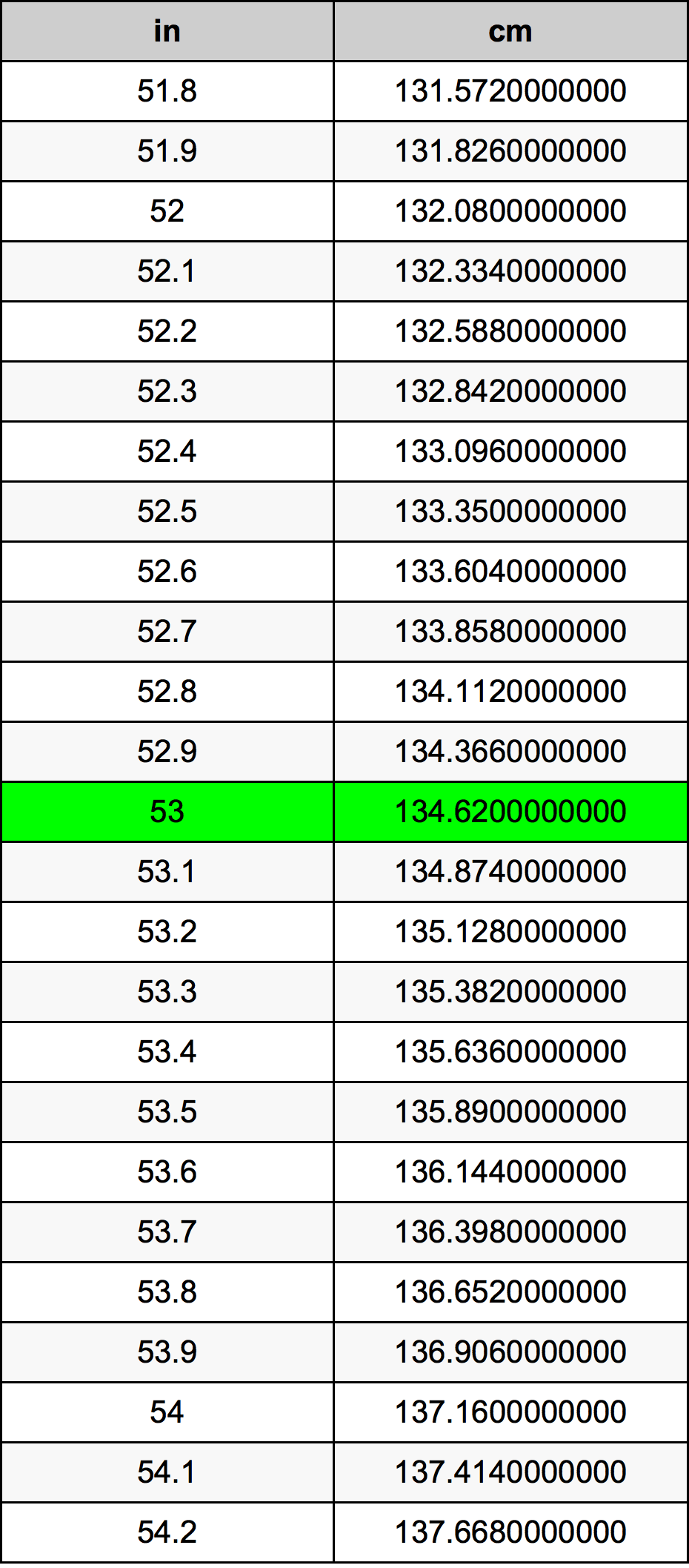Inches To Centimeters

# 53 in to cm53 Inches to Centimeters

in
=
cm

## How to convert 53 inches to centimeters?

 53 in * 2.54 cm = 134.62 cm 1 in
A common question is How many inch in 53 centimeter? And the answer is 20.8661417323 in in 53 cm. Likewise the question how many centimeter in 53 inch has the answer of 134.62 cm in 53 in.

## How much are 53 inches in centimeters?

53 inches equal 134.62 centimeters (53in = 134.62cm). Converting 53 in to cm is easy. Simply use our calculator above, or apply the formula to change the length 53 in to cm.

## Convert 53 in to common lengths

UnitLengths
Nanometer1346200000.0 nm
Micrometer1346200.0 µm
Millimeter1346.2 mm
Centimeter134.62 cm
Inch53.0 in
Foot4.4166666667 ft
Yard1.4722222222 yd
Meter1.3462 m
Kilometer0.0013462 km
Mile0.0008364899 mi
Nautical mile0.0007268898 nmi

## What is 53 inches in cm?

To convert 53 in to cm multiply the length in inches by 2.54. The 53 in in cm formula is [cm] = 53 * 2.54. Thus, for 53 inches in centimeter we get 134.62 cm.

## 53 Inch Conversion Table## Alternative spelling

53 Inch to cm, 53 Inch in cm, 53 Inches to cm, 53 Inches in cm, 53 Inches to Centimeters, 53 Inches in Centimeters, 53 Inch to Centimeter, 53 Inch in Centimeter, 53 Inches to Centimeter, 53 Inches in Centimeter, 53 Inch to Centimeters, 53 Inch in Centimeters, 53 in to Centimeters, 53 in in Centimeters